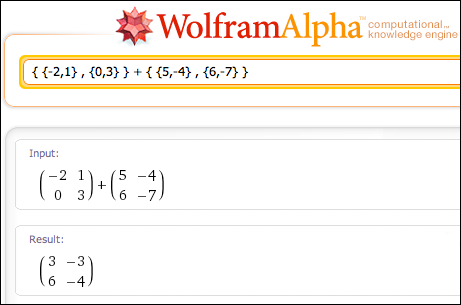﻿ Basic Arithmetic With Matrices

#Basic Arithmetic With Matrices

You may want to review:  Introduction to Matrices

Whenever you get a new mathematical object (like matrices), it's important to develop tools to work with the new object.

In this exercise, you'll learn how to do basic arithmetic operations with matrices: adding, subtracting, and multiplying by a constant.

Matrices can only be added or subtracted when they have the same size. In this situation, you just add/subtract the corresponding entries.

For example:

\begin{align} &\cssId{s11}{\begin{bmatrix} -2 & 1\cr 0 & 3 \end{bmatrix}} \cssId{s12}{+} \cssId{s13}{\begin{bmatrix} 5 & -4\cr 6 & -7 \end{bmatrix}}\cr\cr &\qquad \cssId{s14}{=} \cssId{s15}{\begin{bmatrix} -2+5 & 1+(-4)\cr 0+6 & 3+(-7) \end{bmatrix}}\cr\cr &\qquad \cssId{s16}{=} \cssId{s17}{\begin{bmatrix} 3 & -3\cr 6 & -4 \end{bmatrix}} \end{align}

\begin{align} &\cssId{s18}{\begin{bmatrix} -2 & 1\cr 0 & 3 \end{bmatrix}} \cssId{s19}{-} \cssId{s20}{\begin{bmatrix} 5 & -4\cr 6 & -7 \end{bmatrix}}\cr\cr &\qquad \cssId{s21}{=} \cssId{s22}{\begin{bmatrix} -2-5 & 1-(-4)\cr 0-6 & 3-(-7) \end{bmatrix}}\cr\cr &\qquad \cssId{s23}{=} \cssId{s24}{\begin{bmatrix} -7 & 5\cr -6 & 10 \end{bmatrix}} \end{align}

Precisely, we have:

Suppose matrices $\,A\,$ and $\,B\,$ have the same size.

Then, the sum $\,S=A+B\,$ is defined by: $$\cssId{s30}{s_{ij} = a_{ij} + b_{ij}}$$

The difference $\,D=A-B\,$ is defined by: $$\cssId{s32}{d_{ij} = a_{ij} - b_{ij}}$$

In particular, matrices with different sizes cannot be added or subtracted.

WolframAlpha uses braces $\,\{\ \}\,$ to input matrices, not brackets $\,[\ ]\,.$ Then, it displays the matrices (so you can verify your input) using parentheses $\,(\ )\,.$ Get used to this—different academic disciplines and different environments often use different notation.

The screenshot below shows how to input matrices at wolframalpha.com.

Each row goes inside a pair of braces $\,\{\ \}\,,$ with elements separated by commas, like this: $$\cssId{s45}{\{-2,1\}}$$

The rows themselves are also separated by commas, like this: $$\cssId{s47}{\{-2,1\}}\ \cssId{s48}{\ ,\ } \cssId{s49}{\{0,3\}}$$

The entire matrix is enclosed within a pair of braces, like this: $$\cssId{s51}{\{}\ \cssId{s52}{\{-2,1\}}\cssId{s53}{\ ,\ }\cssId{s54}{\{0,3\}}\ \cssId{s55}{\}}$$

If you want, cut-and-paste the following input to WolframAlpha to duplicate what you see in the image below:

{ {-2,1} , {0,3} } + { {5,-4} , {6,-7} }## Multiplying a Matrix by a Constant

It is equally easy to multiply a matrix by a constant; each entry gets multiplied by the constant.

For example,

\begin{align} &\cssId{s62}{7} \cssId{s63}{\begin{bmatrix} -2 & 1\cr 0 & 3 \end{bmatrix}}\cr\cr &\qquad \cssId{s64}{=} \cssId{s65}{\begin{bmatrix} 7(-2) & 7(1)\cr 7(0) & 7(3) \end{bmatrix}}\cr\cr &\qquad \cssId{s66}{=} \cssId{s67}{\begin{bmatrix} -14 & 7\cr 0 & 21 \end{bmatrix}} \end{align}

Precisely, we have:

DEFINITION multiplying a matrix by a constant

Let $\,M\,$ be a matrix, with members $\,m_{ij}\,.$ Let $\,k\,$ be a real number.

Then, the new matrix $\,kM\,$ has the same size as $\,M\,$; the member in row $\,i\,$ and column $\,j\,$ of $\ kM\$ is $\ k\,m_{ij}\ .$

Note: The real number multiplier is often called a constant or a scalar.

When working with matrices, it's important to distinguish between the real number $\,0\,$ and a zero matrix. To help with this distinction, we define $\,0_{m\times n}\,$ (zero, with a subscript of $\,m\times n\,$) to mean the zero matrix of size $\,m\times n\,.$

You can read $\,0_{m\times n}\,$ aloud as ‘the $\,m\,$ by $\,n\,$ zero matrix’.

Thus, if $\,A\,$ is a $\,2\times 3\,$ matrix, then: $$\cssId{s90}{A-A} \cssId{s91}{=} \cssId{s92}{0_{2\times 3}}$$

Or, if $\,A\,$ is a $\,p\times q\,$ matrix, then: $$\cssId{s94}{0A} \cssId{s95}{=} \cssId{s96}{0_{p\times q}}$$

Be aware that many advanced textbooks write simple things like $\,A-A=0,\,$ knowing that the audience has enough mathematical maturity to realize that the zero is really the zero matrix with the same size as $\,A\,.$ However, in this exercise, we will be careful to distinguish between the real number zero, and a zero matrix.

## Examples

Let   $A = \left[ \begin{smallmatrix} 2 & -1\cr 0 & 3\end{smallmatrix} \right] \,,$   $B = \left[ \begin{smallmatrix} -3 & -5\cr 1 & 0\end{smallmatrix} \right] \,,$   and   $C = \left[ \begin{smallmatrix} 0 & 1 & 1\cr 2 & -1 & 0\end{smallmatrix} \right] \,.$

Then:

\begin{align} &\cssId{s109}{A+2B}\cr\cr &\qquad \cssId{s110}{=} \cssId{s111}{\begin{bmatrix} 2 & -1\cr 0 & 3 \end{bmatrix}} \cssId{s112}{+} \cssId{s113}{2} \cssId{s114}{\begin{bmatrix} -3 & -5\cr 1 & 0 \end{bmatrix}} \cr\cr &\qquad \cssId{s115}{=} \cssId{s116}{\begin{bmatrix} 2 & -1\cr 0 & 3 \end{bmatrix}} \cssId{s117}{+} \cssId{s118}{\begin{bmatrix} -6 & -10\cr 2 & 0 \end{bmatrix}} \cr\cr &\qquad \cssId{s119}{=} \cssId{s120}{\begin{bmatrix} -4 & -11\cr 2 & 3 \end{bmatrix}} \end{align}

As a second example, $\,A+C\,$ is not defined, since $\,A\,$ and $\,C\,$ have different sizes.